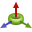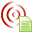# Defining Spherical Modes Data Manually

Define the propagation direction, index scheme and modes to create spherical modes data. Use the spherical modes data definition when defining an equivalent source or receiving antenna.

1. On the Construct tab, in the Define group, click theField/Current Data icon. From the drop-down list selectManually Define Spherical Modes Data.
2. Under Propagation direction, select one of the following:
• To illuminate the model with modes propagating towards $r=0$ , spherical Hankel function of the first kind, ${z}_{N}^{\left(3\right)}={h}_{N}^{\left(1\right)}$ , select Inward.
• To illuminate the model with modes propagating towards $r=\infty$ , spherical Hankel function of the second kind, ${z}_{N}^{\left(4\right)}={h}_{N}^{\left(2\right)}$ , select Outward.
3. In the TE/TM cell, select one of the following:
• TE
The transverse electric mode of propagation (no E-field in the direction of propagation).
TM
The transverse magnetic mode of propagation (no H-field in the direction of propagation).
4. In the Index scheme cell, select one of the following:
• Normal
This scheme uses the traditional smn index. You can specify TE-mode (s = 1) or TM-mode (s = 2) and the indices M and N.

M is the mode index in the azimuth direction $\varphi$ and N is the mode index in the radial direction and must be in the range 1, 2....

Feko does not distinguish between even and odd modes (with $\mathrm{cos}\left(M\phi \right)$ and $\mathrm{sin}\left(M\phi \right)$ angular dependencies), but rather use the angular dependency ${e}^{jM\phi }$ . The index M can also be negative, but it must be in the range −N..N.

Compressed

This scheme uses compressed one-dimensional mode numbering scheme. The J mode index is then specified in the index column. Here

(1) $J=2\left[N\left(N+1\right)+M-1\right]+s$
where s = 1 for TE-modes and s = 2 for TM-modes.

This unified mode numbering scheme allows the computation of an extended scattering matrix (with network and radiation ports). The index J then represents a unique port number in the scattering matrix.

5. In the Mag. sqrt(W) cell, specify the absolute value of the complex amplitude for the spherical mode. Due to the spherical modes normalisation, the amplitude unit is $\sqrt{W}=\sqrt{VA}$ .
6. In the Phase [deg.] field, specify the phase of the complex amplitude for the spherical mode.
7. In the Label field, specify a unique label for the spherical modes data.
8. Click Create to define the spherical modes data and to close the dialog.